# Test: Sets- 2

## 25 Questions MCQ Test Quantitative Aptitude for GMAT | Test: Sets- 2

Description
Attempt Test: Sets- 2 | 25 questions in 50 minutes | Mock test for GMAT preparation | Free important questions MCQ to study Quantitative Aptitude for GMAT for GMAT Exam | Download free PDF with solutions
QUESTION: 1

### Among the respondents of a survey, one-fourth do not like to watch movies; and of those who do not like to watch movies, two-third do not like to read also. Can the number of respondents who do not like to read be 70? (1) The number of respondents who like to read and also like to watch movies is 110 (2) The number of respondents of the survey is 480

Solution:

Steps 1 & 2: Understand Question and Draw Inferences

Given: Let the total # of respondents be T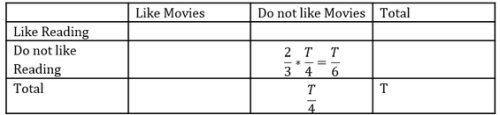To find: Can number of people who don’t like to read be 70?

• Let this number be Z
• The different cells of the table can be filled in terms of T and Z as under: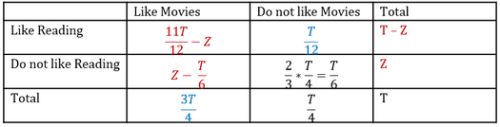Step 3: Analyze Statement 1 independently

The number of respondents who like to read and also like to watch movies is 110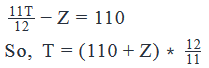Since T is the number of students, it must be a positive integer.

Since Z = 70 doesn’t lead to a positive integer value of T, Z cannot be 70.

Step 4: Analyze Statement 2 independently

The number of respondents of the survey is 480

T = 480

So, the above table becomes: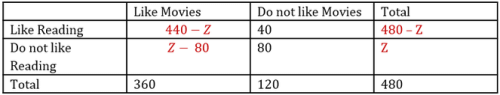Since there are 80 people who do not like reading, Z cannot be less than 80.

Therefore, Z cannot be 70

Step 5: Analyze Both Statements Together (if needed)

Since we’ve already arrived at a unique answer in each of Steps 3 and 4, this step is not required

QUESTION: 2

### If 30 percent of the students in an MBA program had read the work of Shakespeare, 35 percent had read the work of Peter Drucker and 5 percent had read the work of both Shakespeare and Peter Drucker, what percent of the students had read the work of neither Shakespeare nor Peter Drucker?

Solution:

Given:

• The given information may be represented visually as under: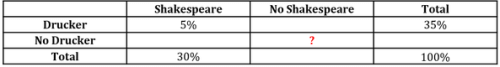To find: % of students who had read neither Shakespeare nor Drucker

Approach:

1. The key to solving this question was really representing the given information visually (using a table as done above or a tree structure). Once this has been done, the question solves pretty easily.
2. We’ll find the values in the relevant unknown cells and complete the table.
1. (% of Students who read No Shakespeare) = (Total Students) – (% of Students who read Shakespeare)
2.  (% of Students who read Drucker but No Shakespeare) = (% of Students who read Drucker) – (% of Students who read Shakespeare and Drucker)
3. So, (% of Students who Read No Drucker and No Shakespeare) = (% of Students who read No Shakespeare) – (% of Students who read Drucker but No Shakespeare)

Working Out:

• Using the equations delineated in Step 2 of the Approach, the values in the relevant unknown cells are found as under (these values are put in blue)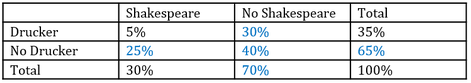Looking at the answer choices, we see that the correct answer is Option D

QUESTION: 3

### Of the 80 children in a group, 64 like chocolates. How many children do not like ice-cream? (1) The number of children who like chocolates but do not like ice-cream is 10 more than the number of children who like ice-cream but do not like chocolate (2) The number of children who like both ice-cream and chocolate is 38 more than the number of children who neither like ice-cream nor like chocolate

Solution:

Steps 1 & 2: Understand Question and Draw Inferences

Given: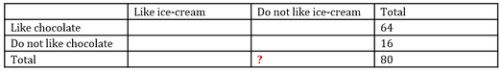To find: Number of children who don’t like ice-cream

Step 3: Analyze Statement 1 independently

The number of children who like chocolates but do not like ice-cream is 10 more than the number of children who like ice-cream but do not like chocolate

Let the number of children who like ice-cream but do not like chocolate be Y

Let’s represent the given information in the table:

• Green color denotes the fact given in Statement 1
• Red color denotes the inferred information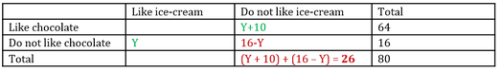Thus, number of students who do not like ice-cream is 26.

Sufficient.

Step 4: Analyze Statement 2 independently

The number of children who like both ice-cream and chocolate is 38 more than the number of children who neither like ice-cream nor like chocolate

Let the number of children who neither like ice-cream nor like chocolate be X

Let’s represent the given information in the table:

• Green color denotes the fact given in Statement 2
• Red color denotes the inferred information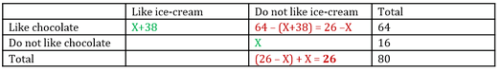Thus, number of students who do not like ice-cream is 26.

Sufficient.

Step 5: Analyze Both Statements Together (if needed)

Since we’ve already arrived at a unique answer in each of Steps 3 and 4, this step is not required

QUESTION: 4

Out of the 60 employees of a startup, only 10 are non-engineers. How many employees of the startup are engineers with a MBA degree?

(1) Only 1 out of every 12 employees with a MBA degree is a non-engineer

(2) More than 55 percent of all employees have a MBA degree

Solution:

Steps 1 & 2: Understand Question and Draw Inferences

Given: 60 employees classified on 2 attributes:

• Engineer/Non-Engineer
• MBA Degree/ No MBA Degree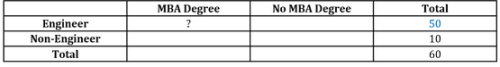• (Number of Non-engineers) = 10
• So, (Number of Engineers) = 60 – 10 = 50
• Note: In the above table and the other tables in this solution, black font color is used to denote the given information, whereas blue font color is used to denote the inferred information

To find:  Number of Engineers with MBA Degree

Step 3: Analyze Statement 1 independently

Statement 1 says that ‘Only 1 out of every 12 employees with a MBA degree is a non-engineer’

• Let the total number of employees with a MBA degree be x
• x≤60
• So, (Number of non-engineers with a MBA degree) = x/12
• Since number of people must be an integer, x must be a multiple of 12
• We have already seen that x≤60
• So, multiples of 12 that are less than equal to 60 are: {12, 24, 36, 48, 60}
• Therefore, (Number of engineers with a MBA degree) = 11x/12
• 11x/12

must be less than equal to the row total of 50

• Out of {12, 24, 36, 48, 60}, we can reject the value 60 on this basis (because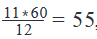, which is greater than the row total of 50)
• So, possible values of x = {12, 24, 36, 48}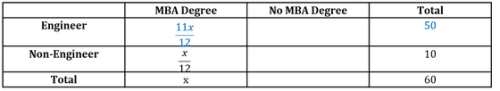• Since we do not know still know a unique value of x, Statement 1 is not sufficient

Step 4: Analyze Statement 2 independently

Statement 2 says that ‘More than 55 percent of all employees have a MBA degree’

• Let the total number of employees with a MBA degree be x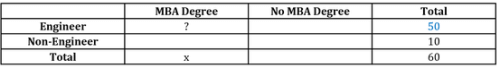• So, x > 55% of 60
• That is, x > 33

However, this doesn’t answer the question about number of Engineers with MBA Degree

Not Sufficient.

Step 5: Analyze Both Statements Together (if needed)

• From Statement 1:
• x = {12, 24, 36, 48}
• From Statement 2, x > 33
• Combining the 2 statements, we get that:
• x = {36, 48}
• So, the 2 statements together are also not sufficient to answer the question

QUESTION: 5

In a certain town, 40 percent of the residents own a car. What percent of the residents own neither a house nor a car?

(1) Each resident who owns a house also owns a car but the vice-versa is not true

(2) 30 percent of the residents own a house

Solution:

Steps 1 & 2: Understand Question and Draw Inferences

The residents of the town are classified according to two attributes – housing and car ownership.

Let’s depict this fact, and the given information in a table.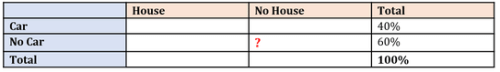Step 3: Analyze Statement 1 independently

So, the percentage of people who own a house but not own a car = 0%

Therefore, the percentage of people who neither own a house nor own a car = 60% – 0% = 60%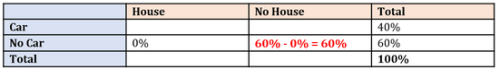Therefore, St. 1 is sufficient to answer the question.

Step 4: Analyze Statement 2 independently

Statement 2 says that 30% of all residents own a house. Let’s represent this information in the table: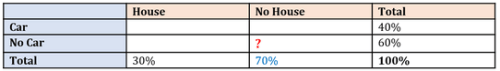Not sufficient to determine a unique value of the unknown.

Step 5: Analyze Both Statements Together (if needed)

Since we arrived at an answer in Step 3, this step is not required.

QUESTION: 6

In a class of 120 students, 80 enrolled for a Mathematics seminar, 60 enrolled for Business Basics seminar, while 12 did not enroll for either of the 2 seminars. How many students enrolled for both the seminars?

Solution:

Given: A class of 120 students who are classified on their enrollment for 2 seminars:

• Mathematics – Enrolled/ Did not Enroll
• Business Basics – Enrolled/ Did not Enroll

Representing the given information visually: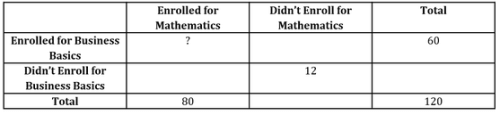TO find: Number of students who enrolled for both seminars

Approach:

1. We’ve already done the first step of the approach by representing the given information visually
2. Now, we will complete the table to get to the answer

Working Out

• (Number of students who didn’t enroll for mathematics) = 120 – 80 = 40
• So, (Number of students who Enrolled for BB – Didn’t enroll for Mathematics) = 40 – 12 = 28
• (Number of students who enrolled for both seminars) = 60 – 28 = 32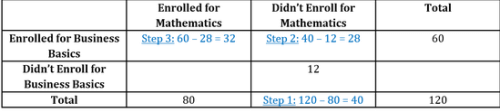QUESTION: 7

List A ={1, 2, 3, 4, 5, 6, 7, 8, 9, 10}

The integers from list A are used to form two distinct sets P and Q each consisting of distinct integers only. If all the integers from list A are used at least once and none of the integers are used more than twice, how many integers from list A are used more than once?

1) The numbers of integers in sets P and Q are, respectively, 2 and 3 less than the number of integers in list A.

2) The difference between the sum of the integers in sets P and Q and the sum of the integers in list A is 15.

Solution:

Steps 1 & 2: Understand Question and Draw Inferences

Given:

• List A:{1,2,3…9,10}, a total of 10 integers

• Let the total number of integers in set P be x
• Let the integers used once from list A = a
• Let the integers used twice from list A = b

• Let the total number of integers in set Q be y
• Let the integers used once from list A = c
• Let the integers used twice from list A = d

To Find: Number of integers used more than once

• Number of integers used more than once = Number of integers used two times
• So number of integers used two times = Total number of integers in set P and set Q – number of integers used one time
• So, b+d = (x+y) – (a+b)
• Since all integers are used atleast once,
• so a+b =10
• So, b+d = (x+y)-10

So to determine the number of integers used more than once, we will have to find value of (x+y).

Step 3: Analyze Statement 1 independently

The numbers of integers in sets P and Q are, respectively, 2 and 3 less than the number of integers in list A.

• So, Total Integers in set P = x = 10 – 2 = 8
​So, Total Integers in set Q = y = 10 – 3 = 7

• x + y = 15

Since we know the value of x+y, we will find the answer.

Step 4: Analyze Statement 2 independently

The difference between the sum of the integers in sets P and Q and the sum of the integers in list A is 15.

• Sum of integers in list A = 1+2+3….+10 = S, say

• Sum of integers in sets P and Q = 1+2+3…+10 + Sum of repeated integers

• Difference in sum of the integers in sets P and Q = S + sum of repeated integers – S = Sum of repeated integers

• Sum of Repeated integers = 15

• So, the sum of repeated integers 15 can be expressed as a sum of integers in various ways.
• For ex: (9, 6) gives the sum as 15 and number of repeated integers =2
• or (4, 5, 6) gives the sum as 15 and number of repeated integers = 3

Since we do not know the exct number of repeated integers, we will not be able to determine the value of x &y

Step 5: Analyze Both Statements Together (if needed)

As we have a unique answer from step-3, this step is not required.

QUESTION: 8

At a certain university, 120 students were asked to take a surprise quiz to check their awareness of history and civil rights. The score distribution of the students is tabulated below: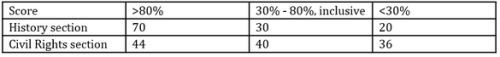If 70 students scored greater than 30% in both tests, what was the number of students who scored less than 30% in both the history and the civil rights sections of the quiz?

Solution:

Given:

• Total number of students = 120
• Score distribution of these 120 students in History and Civil Rights is as shown in the table
• Number of students who scored ≥ 30% in History and ≥30% in Civil Rights = 70

To find: Number of students who scored <30% in History and <30% in Civil Rights

Approach:

1. In the table of scores given to us, the scores of the students are divided in 3-categories: > 80%, 30% - 80%, inclusive, and <30%
• But upon reading the question, we realize that in order to answer the question, we need to consider only 2 categories of scores:
• ≥ 30% (includes students who scored > 80% and between 30% - 80%, inclusive)
• < 30%
2. Now, there are 2 subjects (History and Civil Rights), and 2 score-categories to be considered (≥ 30%, < 30%)
• So, we’ll solve this question by drawing a table as under: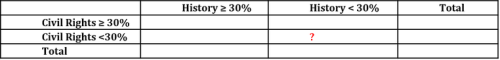• Now, using the given information, we’ll fill this table and work towards arriving at the answer

Working Out:

• Filling the given information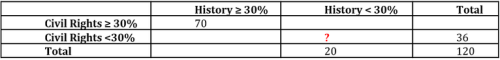Note that from the table given in the question, we know that the total number of students who scored less than 30% in Civil Rights is 36, and the total number of students who scored less than 30% in History is 20

• Step 1: The total number of students who scored ≥ 30% in:
• History = 120 – 20 = 100
• Civil Rights = 120 – 36 = 84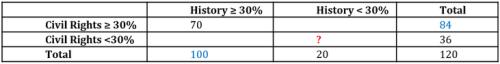Step 2:  The number of students who scored < 30% in History and ≥ 30% in Civil Rights

• = 84 – 70 = 14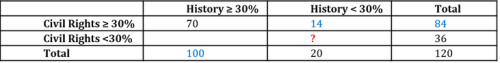Step 3: The number of students who scored < 30% in History and in Civil Rights

• = 20 – 14
• = 6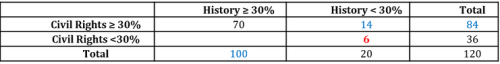• Thus, our required value is 6

Looking at the answer choices, we see that the correct answer is Option A

QUESTION: 9

200 students in an MBA program voted for 2 electives A and B to be included in their curriculum. A student could vote for either one or both or none of the electives. If 30% of the students voted for elective A, how many students voted for both the electives?

1) The sum of the number of students who voted for none of the electives and those who voted for only elective A is 50% more than the number of students who voted for only elective B.

2) 60% of the students did not vote for elective B.

Solution:

Steps 1 & 2: Understand Question and Draw Inferences

Given:

• Total number of students who voted = 200

• Let us assume :
• a be the number of students who voted only for elective A.
• b be the number of students who voted both for elective A and elective B.
• c be the number of students who voted only for elective B.
• d be the number of students who did not vote for any elective.

• This is represented in the venn diagram below: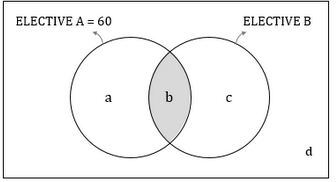• So, we will have a + b + c + d = 200……(1)

• 30% students voted for elective A
• Students who voted for elective A = 30%*200 = 60
• So, a + b = 60 ….(2)

To Find: Value of b

Step 3: Analyze Statement 1 independently

The sum of the number of students who voted for none of the electives and those who voted for only elective A is 50% more than the number of students who voted for only elective B.

• a + d = 1.5c ....(3)
• Now, since we have 4 unknowns and 3 equations, we will not be able to determine a unique value of b.

Step 4: Analyze Statement 2 independently

60% of the students did not vote for elective B.

• a + d = 60% of 200 = 120
• Now, since we have 4 unknowns and 3 equations, we will not be able to determine a unique value of b.

Step 5: Analyze Both Statements Together (if needed)

1. From statement 1, a+d =1.5c
2. From statement 2, a+d =120

Now we have 4 equations and 4 unknowns, so we will be able to determine a unique value of b.

QUESTION: 10

Out of the 14 people in a group, 3 like to play Badminton, Soccer and Volleyball. If there are 7 people in the group who like to play Volleyball and 5 who like to play Badminton and Soccer, what is the number of people who do not like to play any of the 3 games?

Solution:

Given: 14 people classified for their liking for games as follows: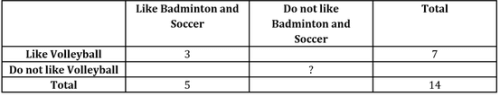To find: (Number of people who Do not Like Volleyball and who do not Like Badmintion and Soccer = Number of people who Do Not Like Volleyball – Number of people who Like Badminton and Soccer)

Approach:

1. We’ve already done the first step of the approach by representing the given information visually
2. Now, we will complete the table to get to the answer

Working Out

1. In the following tables, black font represents the given information while blue font represents deduced information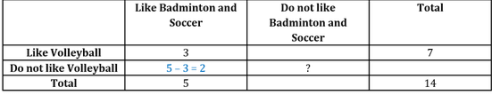• By focusing on the ‘Like Badminton and Soccer’ column, we can find that ‘Do not Like Volleyball – Like Badminton and Soccer’ number is 5 – 3 = 2

2. Now, let’s focus at the last column (‘Total’) of the table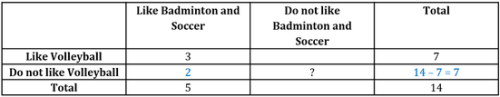‘Do Not Like Volleyball’ Total number = 14 – 7 = 7

3. Finally, let’s focus on the ‘Do not Like Volleyball’ row of the table: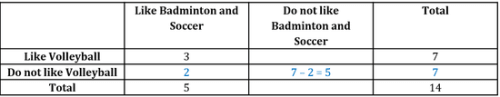‘Do not Like Volleyball –  Like Badminton and Soccer’ Number = 7 – 2 = 5

This is our required number

QUESTION: 11

Set A contains n distinct positive integers, all greater than 1. If the number of even integers in the set is 5 and the set also contains 4 odd prime numbers, what is the value of n?

(1) The set contains 2 odd composite numbers.

(2) The set contains 6 composite numbers

Solution:

Steps 1 & 2: Understand Question and Draw Inferences

Given: A set of n distinct positive integers, all greater than 1,is classified based on 2 attributes:

• Even/Odd nature
• Prime/Composite nature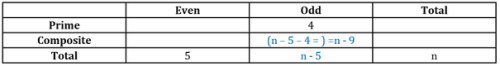• (In this and the forthcoming tables in this solution, black font color denotes given information and blue font color denotes deduced information)

To find:  n = ?

Step 3: Analyze Statement 1 independently

Statement 1 says that ‘The set contains 2 odd composite numbers’

• So, n – 9 = 2
• Therefore, n = 11
• Statement 1 is sufficient to find the value of n

Step 4: Analyze Statement 2 independently

Statement 2 says that ‘The set contains 6 composite numbers’

• Let’s represent this information in the table and complete the table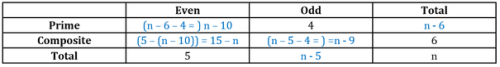Completing the table, we see that we still do not get the value of n

So, Statement 2 alone is not sufficient

Step 5: Analyze Both Statements Together (if needed)

Since we’ve already arrived at a unique answer in Step 3, this step is not required

QUESTION: 12

From a list of integers, all the multiples of 5 are sorted into Set A and all the even integers are sorted into Set B. If 60% of the integers in Set A and 50% of the integers in Set B are not divisible by 10, which of the following statements must be true?

I. The number of integers in Set A is less than the number of integers in Set B

II. The number of integers in Set B that are divisible by 10 is greater than the corresponding number in Set A

III. The number of odd multiples of 5 in Set A is greater than the number of integers in Set B that are not divisible by 10.

Solution:

Given:

• Within a list of integers:
• Set A contains multiples of 5
• Let the total number of integers in Set A be a
• 60% of these a multiples of 5 are not divisible by 10 (= 2*5)
• That is, 0.6a multiples of 5 are not divisible by 2 (hence, are odd)
• And, the remaining 0.4a multiples of 5 are even
• Set B contains even integers
• Let the total number of integers in Set B be b
• 50% of these b even integers are not divisible by 10 (= 2*5)
• That is, 0.5b even integers are not multiples of 5
• And, the remaining 0.5b even integers are multiples of 5
• Representing the given and inferred information in a Venn Diagram: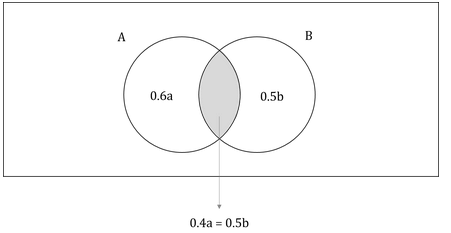To find: Which of the 3 statements must be true?

Approach:

1. Upon reading the 3 statements, we see that to evaluate them, we first need to know the actual value of a and b, or the value of a in terms of b.
2. Once we have a relation between a and b, we’ll evaluate the 3 statements one by one to see which is true without exceptions (that is, true for all values of a and b)

Working Out:

• Finding a relation between a and b
• From the Venn Diagram, we notice that 0.4a = 0.5b
• That is, a=5b/4
• Evaluating Statement I
• The number of integers in Set A is less than the number of integers in Set B
• Statement I says that a < b
• However, since a=5b/4, a>b
•
• So, clearly, Statement I is false

• Evaluating Statement II
• The number of integers in Set B that are divisible by 10 is greater than the corresponding number in Set A
• (Number of integers in Set B that are divisible by 10) = (Overlap zone between Sets A and B) = (Number of integers in Set A that are divisible by 10)
• So, Statement II is false

• Evaluating Statement III
• The number of odd multiples of 5 in Set A is greater than the number of integers in Set B that are not divisible by 10
• Statement III says that 0.6a > 0.5b
• Now, we’ve determined that a=5b/4
• This means, 0.6a=0.6 ∗ 5b/4 = 3b/4 = 0.75b
• Since b is a positive number, 0.75b will be greater than 0.5b
• Therefore, 0.6a is indeed greater than 0.5b
• Hence, Statement III is true for all values of a and b

• Determining the correct answer choice
• Thus, we’ve determined that only Statement III is a must be true statement

Looking at the answer choices, we see that the correct answer is Option C

QUESTION: 13

A class in a certain school consists of boys and girls in the ratio of 2:1 . In a mathematics test, 60% of the class passed the test. If 40% of the boys failed the test, what percentage of the class are girls who passed the test?

Solution:

Given

• Let the number of boys be 2x and number of girls be x
• Total students = 3x
• Number of students who passed the test = 60% of 3x = 1.8x
• Number of boys who failed the test = 40% of 2x = 0.8x

To Find:

Approach

1. As we know the number of students in the class = 3x, we need to express the number of girls who passed the test in terms of x.
2. Number of students who passed the test = Number of boys who passed the test + Number of girls who passed the test
a.  We know the number of students who passed the test, so we need to find the number of boys who passed the test
3. As we know the number of boys in the class and the number of boys who failed the test, we can find the number of boys who passed the test.

Working Out

1. Number of boys in the class = 2x
1. Number of boys who failed the test = 0.8x
2. So, number of boys who passed the test = 2x – 0.8x = 1.2x
2. Number of students who passed the test = 1.8x
1. Number of boys who passed the test = 1.2x
2. So, number of girls who passed the test = 1.8x – 1.2x = 0.6x
3. So,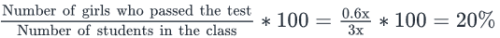So, the number of girls who passed the test are equal to 20% of the total class strength.

QUESTION: 14

A survey was conducted among a group of 83 American travel enthusiasts to determine the countries that the group members had visited. The data collected in the survey is summarized as below:

• 8 had visited Australia and Belgium
• 12 had visited Belgium and Chile
• 4 had visited Australia and Chile
• 14 had visited none of the three countries

What is the number of surveyed travel enthusiasts who had visited all the three countries?

Solution:

Given:

• Total surveyed people = 83
• Data about their visits to 3 countries is provided: Australia (referred to as A in the solution), Belgium (B) and Chile (C)

To find: The number of surveyed people who visited all 3 countries.

Approach:

1. Let the number of surveyed people who visited all 3 countries be x
2. Now, since this is a question that involves 3 sets (A, B and C), the most efficient way to solve it will be Venn Diagram. So, we’ll represent the given information in a Venn Diagram. Since x is the only unknown quantity in this question, we’ll be able to get a linear equation in x and so, get a unique value of x.

Working Out: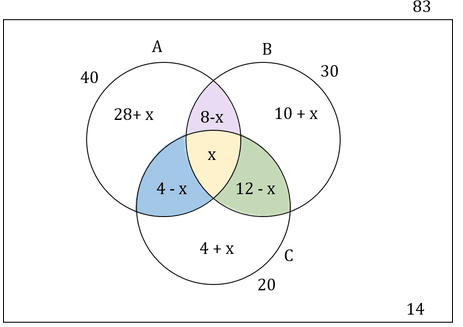• Since total number of people is 83, we can write:
• {(28+x) +(8-x) +x+(4-x)} + {(10+x) + (12-x)} + (4+x) + 14 = 83
• So, 40 + 40 + x = 83
• 80 + x = 83
• Therefore, x = 3

Looking at the answer choices, we see that the correct answer is Option D

QUESTION: 15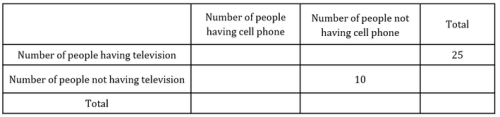The table above shows the result of a survey done on 45 people in a residential area. 25 people in the survey had a television and 10 people had neither a television nor a cell phone.. If the number of people who did not have a television was equal to the number of people who had a television but did not have a cell phone, what was the ratio of the number of people who did not have a cell phone and the number of people who did not have a television?

Solution:

Given

• Number of people who were surveyed = 45
• Number of people who had a television = 25
• Number of people who neither had a television nor a cell phone = 10
• (Number of people who did not have a television) = (Number of people who had a television but did not have a cell phone)

To Find: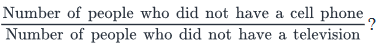• Let this ratio be b

Approach

1. To find the value of b, we need to find:
1. Number of people who did not have a cell phone
2. Number of people who did not have a television
2. Number of people not having a television
1. Let’s assume the number of people not having a television but having a cell phone to be x
1. So, number of people not having a television = 10 + x
3. Also, let’s assume the number of people having a television and a cell phone be y
1. So, number of people having a television but not having a cell phone = 25 – y
4. Number of people not having a cell phone
1. Also, the number of people who do not have a cell phone = x + y
2. Number of people who do not have a cell phone = 35 – y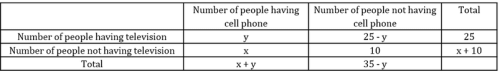• So, total number of people = 25 + (x+ 10) = 35 + x
• Since, we know that total number of people = 45, we will use this relation to find the value of x
• Also, since we know that
1.  Number of people who did not have a television = Number of people who had a television but did not have a cell phone
2. We wil use the above relation to find the value of y and hence the value of b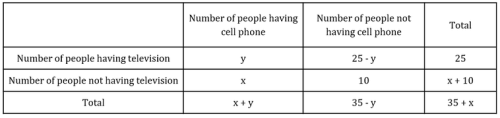Working Out

1. Total number of people = 35 + x = 45
1. So, x = 10
2. So, number of people who did not have a television = x + 10 = 20
3. Number of people who had a television but did not have a cell phone = 25 – y
4. So, 20 = 25 – y
1. y = 5
5. Hence, number of people who did not have a cell phone = 35 – y = 30
6. Number of people who did not have television = x + 10 = 20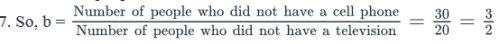QUESTION: 16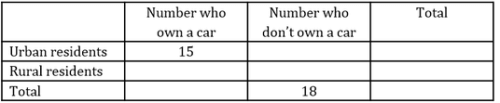The table above shows the research data gathered from a group of rural and urban residents. What is the number of rural residents who own a car?

(1) The number of rural residents in the group is 60% of the number of urban residents in the group.

(2) The difference between the number of urban residents who own a car and the number of rural residents who own a car is one-fifth of the number of rural and urban residents in the group.

Solution:

Steps 1 & 2: Understand Question and Draw Inferences

Given: Data on two parameters are:

• Car ownership – yes or no
• Residence – urban or rural

To find: Number of rural residents who own a car

• Let this number be Q
• Let the number of urban residents without a car be P

The table can be filled in terms of P and Q as follows: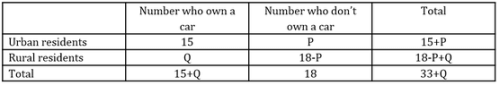Step 3: Analyze Statement 1 independently

• Number of rural residents = 60/100

*(Number of urban residents)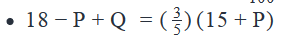• Linear equation with 2 unknowns. Not sufficient to find Q.

Step 4: Analyze Statement 2 independently

• |Number of car-owning urban residents - Number of car-owning rural residents| = 1/5 (Total Number of rural and urban residents)
• |15 - Q| = 15 ∗ (33 + Q)
• Note: we have considered the absolute value of the difference here because we do not know yet which of the 2 values is greater, 15 or Q

Case 1: Q < 15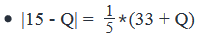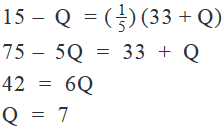Case 2: Q > 15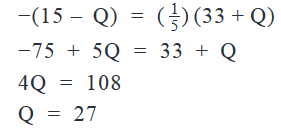2 values of Q. Not sufficient.

Step 5: Analyze Both Statements Together (if needed)

• From Statement 1: 18 - P + Q = 0.6(15 + P). . . (I)
• From Statement 2: Q = 7 or 27
• Let’s put the 2 values of Q in Eq. I to see if both lead to sensible values of P:

Case 1 : Q = 7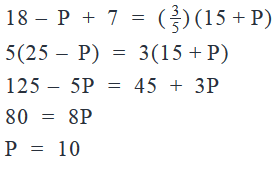Case 2: Q = 27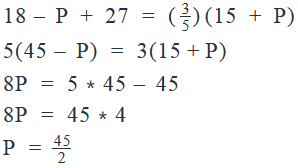Since the number of people cannot be in fractions, Case 2 can be rejected

So, Q = 7.

QUESTION: 17

Of the 48 students in a class, 16 like to study History. What percentage of the girls in the class do not like to study History?

(1) One-third of the boys in the class like to study History

(2) The number of girls who like History is 50 percent of the number of girls who do not like History

Solution:

Steps 1 & 2: Understand Question and Draw Inferences

Given: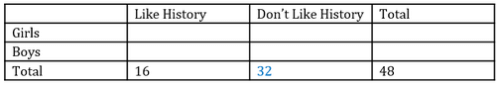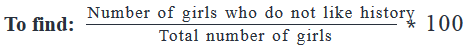• Let number of girls who do not like history = X
• Total number of girls = Y
• We need to find the value of X/Y
• Completing the above table in terms of X and Y: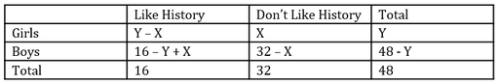Step 3: Analyze Statement 1 independently

One-third of the boys in the class like to study History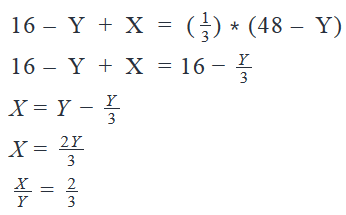Sufficient

Step 4: Analyze Statement 2 independently

The number of girls who like History is 50 percent of the number of girls who do not like History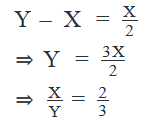Sufficient

Step 5: Analyze Both Statements Together (if needed)

Since we’ve already arrived at a unique answer in Step 3 and Step 4, this step is not required

QUESTION: 18

All the employees of Company X were appraised at the year end. 40% of the employees got a promotion, 50% of the employees got a salary hike and 30% of the employees neither got a promotion nor got a salary hike. If there were 40 employees who got both the promotion and the salary hike, what was the number of employees in the company?

Solution:

Given

• Percentage of employees who got a promotion = 40%
• Percentage of employees who got a salary hike = 50%
• Percentage of employees who neither got a promotion nor a salary hike = 30%
• Number of employees who got both the promotion and the salary hike = 40

To Find: Number of employees in the company?

Approach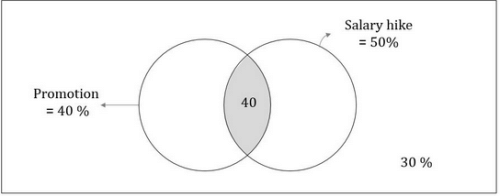1. To find the total number of employees in the company, we will try to express the number of employees who got both the promotion and the salary hike(i.e. 40), as a percentage of the total employees of the company.

Total employees in the company = Employees who got only salary hike + Employees who got only promotion + Employees who got salary hike and promotion + Employees who got neither salary hike nor promotion

1. Adding “Employees who got salary hike and promotion on both sides” we have
2. Total employees in the company + Employees who got salary hike and promotion = (Employees who got only salry hike + Employees who got salary hike and promotion)+ (Employees who got only promotion + Employees who got salary hike and promotion) + Employees who got neither salary hike nor promotion
3. Total employees in the company + Employees who got salary hike and promotion = Employees who got a salary hike+  Employees who got a promotion  + Employees who got neither salary hike nor promotion
4. From the above equation, we can find the percentage of employees who got salary hike and promotion

Working Out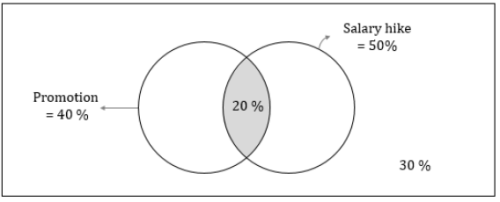1. Total employees in the company + Employees who got salary hike and promotion = Employees who got a salary hike+  Employees who got a promotion  + Employees who got neither salary hike nor promotion
a.  100% + Employees who got salary hike and promotion = 50% + 40% + 30%
b.  Employees who got salary hike and promotion = 20%

2. Now, we know that employees who got salary hike and promotion = 40
1. So, if 20% is 40, 100% = 200

Hence, the total number of employees in the company = 200

QUESTION: 19

The human resources manager of a company compiled the data that the company had on the academic qualifications of its 100 employees. He observed that employees who had an Engineering and an MBA degree were the most in number and employees who had neither an Engineering nor an MBA degree were the least in number. If there were 20 employees who had an Engineering degree, but not an MBA degree, which of the following cannot be the number of employees who had an MBA degree?

Solution:

Given

• Number of employees = 100

• Let us assume:
• Number of employees who only have an MBA degree = c.
• Number of employees who have both an MBA and an Engineering degree = b.
• Number of employees who do neither have an MBA nor an Engineering degree = d.

• Employees having neither Engineering nor an MBA were the least in number.
• So d is the least in the number out of 20,b, c & d.

• Number of employees who had an Engineering degree and not an MBA degree = 20

To Find: Among the options, which of them cannot be the number of employees who had an MBA degree?

Approach

1. Let’s first draw the venn diagram to understand the information given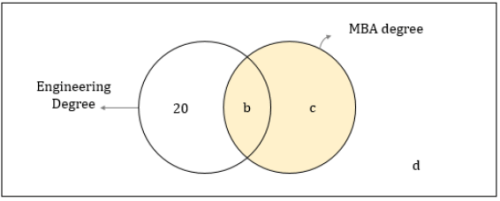2. We need to find the possible range of values of employees who had an MBA degree = b + c

1. So, we need to find the maximum and minimum value of b + c

3. As we know that total number of employees is 100 and d is minimum number of employees possible, we will use this information to find out the range of values of b + c

Working Out

1. As the total number of employees = 100
1. 20 + b + c + d = 100
2. b + c + d = 80

2. Maximum value of b + c
1. Maximum value of b + c is possible, when d is minimum
2. As the minimum value of d = 0, maximum value of b + c = 80…….(1)

3. Minimum value of b + c
1. b + c will be minimum , when d is maximum
2. Since we know the number of employees who had only an Engineering degree = 20, d < 20
3. So, maximum value of d = 19 (remember that out of 20, b, c and d, we are given that d holds the minimum value. So, d must be less than 20)
4. Hence, minimum value of b + c = 80 – 19 = 61…….(2)

4. Using (1) and (2), we can write 61 ≤ b + c ≤ 80

5. The only value in the options that does not lie in the range is 55, option A

QUESTION: 20

A survey was conducted to find out the number of languages spoken by the 210 employees of a company. It was found that 60 employees did not speak English, 150 employees did not speak German and 170 employees did not speak French. If there were 20 employees who did not speak German or English and 20 employees who did not speak French or English, what was the maximum number of employees who spoke only English? Assume that each of the employees spoke at least one language and no employee spoke any language other than English, French and German.

Solution:

Given

• Number of employees = 210
• Number of employees who did not speak English = 60
• So, number of employees who spoke English = 210 – 60 = 150
• Number of employees who did not speak German = 150
• So, number of employees who spoke German = 210 – 150 = 60
• Number of employees who did not speak French = 170
• So, number of employees who spoke French = 210 – 170 = 40
• Number of employees who did not speak German or English = 20
• So, these employees must speak only French (because it’s given that each employee speaks at least one language)
• Number of employees who did not speak French and English = 20
• So, these employees must speak only German (because it’s given that each employee speaks at least one language)

To Find: Maximum number of employees who spoke only English

Approach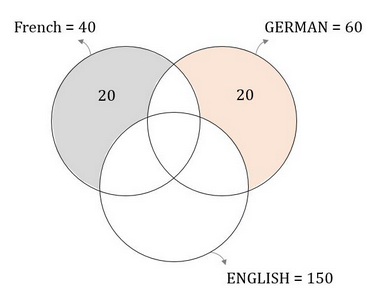1. To find the maximum number of employees who spoke only English, we will first analyse the relation between total number of employees and number of employees who speak only English.
1. Total number of employees = number of employees (who only speak English + who only speak French + who only speak German + who speak both German & French + who speak both German & English + who speak both French & English + who speak all the three languages + who speak none of the languages)
2. Number of employees who only speak English = (Total number of employees) – (number of employees (who only speak French + who only speak German + who speak both German & French + who speak both German & English + who speak both French & English + who speak all the three languages + who speak none of the languages)
3. As we need to maximize the number of employees who spoke only English, we need to minimize the number of employees who spoke English as well as some other language.
4. So, of the employees who speak more one language we would maximize the number of employees who spoke only French and German

2. Now, we know the number of employees who spoke French and German and also the number of employees who spoke only  French and German.

3. We will use this information to maximize the number of employees who spoke only French and German.

Working Out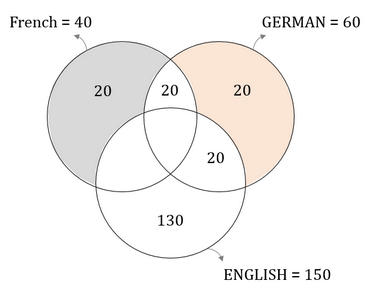1. As there are 40 employees who spoke French and 20 employees who only spoke French,
1. Number of employees who spoke French and some other language = 40 – 20 = 20
2. Similarly, as there are 60 employees who spoke German and 20 employees who spoke only German,
1. Number of employees who spoke German and some other language = 60 – 20 = 40
3. Total number of employees who spoke more than 1 language
1. Number of employees who spoke English + Number of employees who spoke French + Number of employees who spoke German – Total number of employees = 150 + 60 + 40 – 210 = 40
4. As there are 40 employees who spoke more than 1 language, we will try to distribute maximum number of these employees into the number of employees who spoke French and German only.
1. As there are 20 employees who spoke French and some other language and there are 40 employees who spoke German and some other language, the maximum number of employees who spoke French and German = 20
5. So, that still leaves us with 40 – 20 = 20 employees who spoke German and some other language.
1. The only possibility is for these employees to speak German and English
6. So, there are 20 employees who spoke English and some other language
7. As there are 150 employees who spoke English, there can be a maximum of 150 – 20 = 130 employees who spoke only English.

QUESTION: 21

In a certain group of women, 70 percent of the women were employed and 25 percent did not have a graduate degree. Which of the following statements cannot be true?

1. No woman in the group who had a graduate degree was unemployed
2. Less than half of the women in the group were employed and had a graduate degree
3. The number of unemployed women with a graduate degree was 50 percent greater than the number of employed women without a graduate degree
Solution:

Given:

• The Women in the group are divided into groups based on 2 parameters:
• Employment (Employed, Unemployed)
• So, the given information can be represented in a table as follows: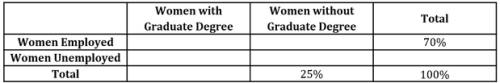• Now, % of women Unemployed = 100% - 70% = 30%
• And, % of Women with Graduate Degree = 100% - 25% = 75%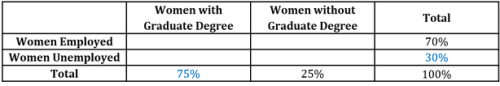To find: Which of the 3 statements cannot be true?

• That is, which of the 3 statements are definitely false

Approach:

1. We’ll evaluate the 3 statements one by one to evaluate which of them are definitely false.

Working Out:

• Evaluating Statement I
• No woman in the group who had a graduate degree was unemployed
• Let the Women with Graduate Degree who are Unemployed be X, and let the Women with Graduate Degree who are Employed be Y.
• As per this Statement, X = 0%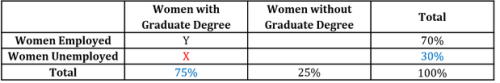• Now, the maximum possible value of Y is 70% (happens when ALL employed women have graduate degrees)
• So, the minimum possible value of X is 5% (since the sum of Y and X is 75%)
• Therefore, Statement I is definitely false.
• Evaluating Statement II
• Less than half of the women in the group were employed and had a graduate degree
• Again, Let the women who were employed and had Graduate Degree be Y
• As per this Statement, Y < 50%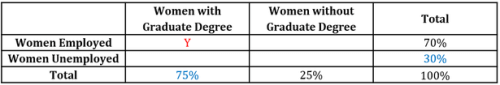Remember that the question is asking if a Statement is definitely false (that is, false for all possible values of Y)

• In our analysis of Statement I above, we saw that the maximum possible value of Y is 70%
• Therefore, for the maximum value of Y, Statement II is false
• But we cannot say at this point if Statement II is definitely false (that is, false for all possible values of Y)
• So, let’s now evaluate is also false for the minimum value of Y.
• The maximum number of Employed Women without Graduate Degree is 25% (happens when ALL women without Graduate Degree are Employed)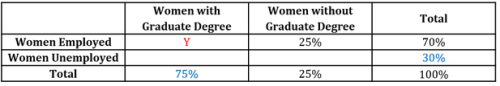• So, the minimum value of Y is 70% - 25% = 45%
• We observe that for the minimum value of Y, Statement II is true.
• Thus, Statement II is true for some values of Y and false for other values of Y.

• So, Statement II is not definitely false.

• Evaluating Statement III
• The number of unemployed women with a graduate degree was 50 percent greater than the number of employed women without a graduate degree
• Let the Women with Graduate Degree who are Unemployed be X, and let the Women without Graduate Degree who are Employed be Z.
• As per Statement III, X = 1.5Z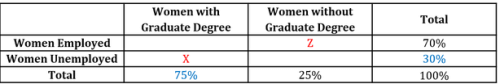• Note that this Statement will be definitely false if no valid pair of (X,Z) satisfies the equation X = 1.5Z
• We will first find the values of X and Z that will satisfy this equation as well as the table above. Then, we will check if these values are acceptable.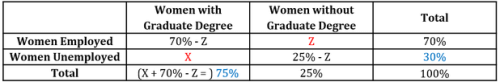• So, the equation that we can write to relate X and Z is:
• X = 75% - (70% - Z)
• = 5% + Z
• Let’s now substitute X = 1.5Z in this equation:
• 1.5Z = 5% + Z
• So, 0.5Z = 5%
• Therefore, Z = 10%
• Corresponding value of X = 1.5*10% = 15%

• So, X = 1.5Z is satisfied by X = 15% and Z = 10%
• We now need to check if these values of X and Z are acceptable
• The minimum possible value of Z is 0%.
• In this case, the table will look as under: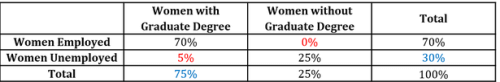The maximum possible value of Z is 25%. In this case, the table will look as under: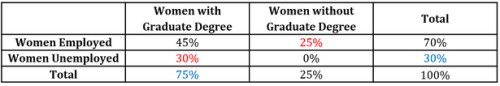• So, the values of Z range from 0% to 25% and correspondingly, the values of X range from 5% to 30%
• So, the values X = 15% and Z = 10% do indeed fall in the acceptable range of values of X and Z.
• So, Statement III is true for one particular pair of X and Z
• Therefore, Statement III is not definitely false

• We’ve seen above that out of Statements I, II and III, only Statement I is definitely false.

Looking at the answer choices, we see that the correct answer is Option A

QUESTION: 22

A group of friends went to an ice-cream parlour and ordered only two types of ice-cream - chocolate and strawberry. Of the people in the group, at least one person ate only one type ice-cream, some people ate both types of ice-cream and at least one person did not eat any type of ice-cream. Did more people eat chocolate ice-cream than strawberry ice-cream?

1) The ratio of the number of people who ate chocolate ice-cream and people who ate strawberry ice-cream was greater than the ratio of the total number of people who went to ice-cream parlour and the number of people who did not eat any type of ice-cream.

2) The number of people who ate only one type of ice-cream is greater than the number of people who ate strawberry ice-cream.

Solution:

Steps 1 & 2: Understand Question and Draw Inferences

Given:

• Let us assume:
• a be the number of people who ate only chocolate ice-cream
• c be the number of people who ate only strawberry ice-cream
• b be the number of people who ate both chocolare & strawberry ice-cream
• d be the number of people who did not eat any of ice-creams

• This is represented in the venn diagram below: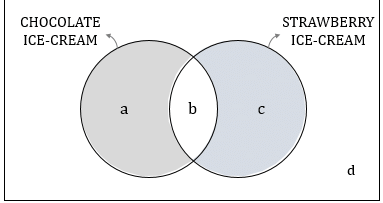• Some people ate both types of ice-cream
• b > 0
• Atleast one person did not eat any type of ice-creamd
• d > 0
• Atleat one person ate only one type of ice-cream
• Either of a or c or both > 0

To Find: If a + b > c + b ?

• If a > c ?

Step 3: Analyze Statement 1 independently

The ratio of the numbers of people who ate chocolate ice-cream and people who ate strawberry ice-cream was greater than the ratio of the total number of people who went to ice-cream parlour and the number of people who did not eat any type of ice-cream.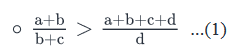• Minimum value of a+b+c+d = a+b+d
• ​Either of a or c >0. So let us take a case, when a>0 and c=0
• ​​​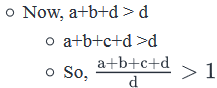• Using (1), we can write: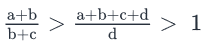• a + b > b + c
• a > c

Step 4: Analyze Statement 2 independently

The number of people who ate only one type of ice-cream is greater than the number of people who ate strawberry ice-cream.

• a + c > b + c• a > b• Does not tell us if a > c.

Step 5: Analyze Both Statements Together (if needed)

As we have a unqiue answer from step-3, this step is not required.

QUESTION: 23

In College X in the United States, there are 200 students in a class, of which  80 are female. If 10% of the class consists of female non-American students and 3/5th of the class are American students, how many of the students in the class are male American students?

Solution:

Given

• Number of students in the class = 200
• Number of female students = 80
• So, number of male students = 200 – 80 = 120
• Number of female non-American students = 10% of 200 = 20
• Number of American students = 3/5 * 200 = 120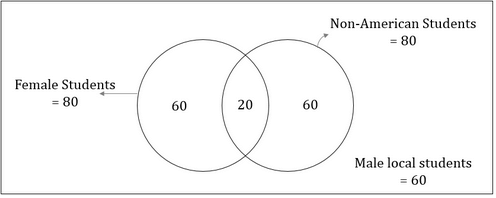To Find: Number of students who are male Americans?

Approach

1. To find the number of male americans, we will use the relation:

1. Number of American students = Number of female American students + Number of male American students
2. We know the number of American students, but we do not know the number of female American students.
3. So, we need to find the number of female American students

2. Now, Number of female students = Number of female American students + Number of female non-American students

1. As we know the number of female students and the number of female non-American students, we can calculate the the number of female American students

Working Out

1. Number of female students = 80
1. Number of female non-American students = 20
2. Number of female American students = 80 – 20 = 60

1. Number of American students = 120
1. Number of female American students = 60 (calculated above)
2. So, Number of male American students = 120 – 60 = 60

So, there are 60 male American students.

QUESTION: 24

In a locality, for every person, who owns only a car, there are 3 people who own only a bike. The number of people who own both a car and a bike is half the number of people who either own only a car or only a bike. If the number of people who neither own a car nor a bike is equal to the number of people who own a bike, which of the following can be the total number of people in the locality?

Solution:

Given

• Let the number of people who own only a car be x
• So, number of people who own only a bike = 3x
• Also, since x denotes the number of people, x must be a non-negative integer
• Number of people who own a car and a bike = ½ *(Number of people who own either a bike or a only car) = ½ * (x+3x) = 2x
• Number of people who neither own a car nor a bike = Number of people who own a bike

To Find: Among the options, which can be the total people in the locality?

Approach

1. We will try to express the number of people in terms of x through the venn diagram and then evaluate the possible options.

Working Out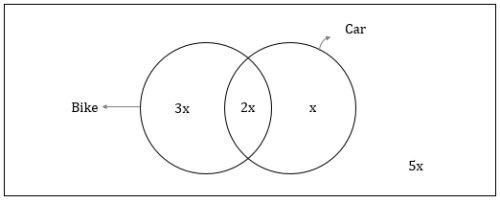1. Number of people who own a bike = 3x + 2 x = 5x
1. So, the number of people who own neither a bike nor a car = 5x
2. Hence, total people in the locality = 3x + 2x + x + 5x = 11x
1. So, the total people in the locality should be a multiple of 11
2. The only multiple of 11 in the options is 66, option C

QUESTION: 25

In a certain class of 66 students, 30 students study Science, 40 students study Humanities and 40 students study Dance. If all the students studied at least one of the subjects, then what is the maximum possible number of students who study all the three subjects?

Solution:

Given:

• Total number of students = 66
• Students who take:
• Science = 30
• Humanities = 40
• Dance = 40
• None of these 3 subjects = 0
• Maximum possible number of students who study all 3 subjects = x

To find: X – N = ?

Approach:

1. To answer the question, we need to find the value of x
2. We’ll visually represent the given information to analyze the maximum possible overlap for 3 subjects.

Working Out: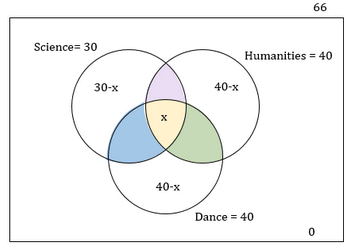• Analyzing the Maximum possible overlap for the 3 subject
• To maximize x, i.e. to minimize the other overlap regions, among the sets, let’s consider the number of students who study only two subjects to be 0
• So, number of students who study only Science = 30 – x
• Number of students who study only Humanities = 40 -x
• Number of students who study only Dance = 40 – x
• So, number of students who study only 1 subject + Number of students who study all the 3 subjects = 66
• (30-x) + (40-x) + (40- x) + x = 66
• 2x = 44
• x = 22Use Code STAYHOME200 and get INR 200 additional OFF Use Coupon Code